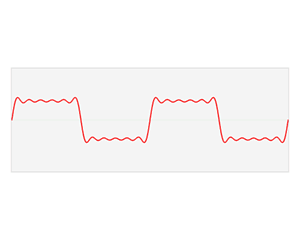# Music Visualizer

The Music Visualizer is a program that uses flocking boids to represent the magnitudes of strong frequencies in different bands of the audible spectrum.

## Boids

The Boids are based on Craig Reynold's flocking algorithm. The boids work by calculating a velocity vector each time the Draw function is called. The velocity is then added to the boids position to create motion.

There are three rules for calculating the velocity vector for each boid:

1. Separate - Boids stay a small distance apart to avoid collisions with neighbors
2. Cohere - Boids fly towards the center of mass of neighbors
3. Velocity - Boids try to match velocity with near boids

Each rule creates a vector which are then summed to create the velocity vector for the boid.

## Fourier

The Fourier transform relates the functions time domain, to the functions frequency domain. The transform allows you to convert a signal from amplitude over time, to magnitude ofer the frequency domain.Red - Time Domain
Blue - Frequency Domain

The Fourier transform is an O(n^2) algorithm but when taken on a discrete set of samples,like digital music, the Fast Fourier Transform can be used. Developed by Cooley-Tukey, the FFT is an O(nlogn) implementation of the Fourier Transform on discrete time intervals.

## Musical visualization

Given the Beets Per Minute of the given song and the frequency domain output of the FFT, the next step is visualizing the music.

My approach for visualizing music with boids is as follows:

1. Calculate interval to separate and join boids to the beat of the song
2. Create a flock of boids to respond to the following frequency intervals:
• 0-300Hz - Bass notes
• 300-600Hz - Mid notes
• 600-1200Hz - Mid High notes
3. weight the amout each flock separated based on the frequency of highest magnitude in each band

### Screens

Mid High Flock
Mid Flock
Low Flock
80pbm Drum Track - The blue (bass) flock has the strongest frequencies thus the largest degree of separation.162bpm Hey Yah! Flocks more evenly distributed130bpm Time to Say Goodbye, during a period of high magnitude low and mid frequencies